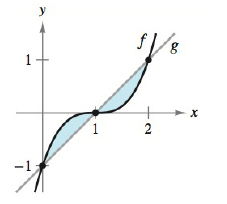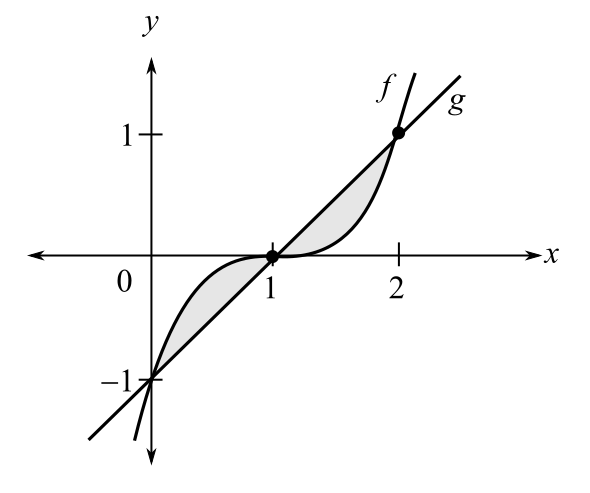Chapter 5.5, Problem 8E### Calculus: An Applied Approach (Min...

10th Edition
Ron Larson
ISBN: 9781305860919

#### Solutions

Chapter
Section### Calculus: An Applied Approach (Min...

10th Edition
Ron Larson
ISBN: 9781305860919
Textbook Problem
15 views

# Finding the Area Bounded by Two Graphs In Exercises 1-8, find the area of the region. See Examples 1, 2, 3, and 4. f ( x ) = ( x − 1 ) 3 g ( x ) = x − 1To determine

To calculate: The area bounded by the region f(x)=(x1)3 and g(x)=x1 in the provided figure,Explanation

Given Information:

The provided function is f(x)=(x1)3 and g(x)=x1 and the provided graph is shown below,

Formula used:

The function f(x) and g(x) are the continuous function on interval [a,b] and g(x)f(x) for all x in [a,b], then, the area bounded by the graphs of f(x) and g(x),x=a and x=b is,

A=ab[f(x)g(x)]dx

Calculation:

Consider the provided function is f(x)=(x1)3 and g(x)=x1.

In the provided function f(x)=(x1)3 and g(x)=x1

Consider the function f(x)=(x1)3 and g(x)=x1 as y=(x1)3 and y=x1.

Now, rewrite the in the form of y.

y3+1=x and y+1=x

Now, from the provided graph, the limit of the function is y=1 and y=1 and the function y+1y3+1

### Still sussing out bartleby?

Check out a sample textbook solution.

See a sample solution

#### The Solution to Your Study Problems

Bartleby provides explanations to thousands of textbook problems written by our experts, many with advanced degrees!

Get Started

#### Find more solutions based on key concepts